# PHP Sessions

Here you will learn all about PHP session from creating session to destroying session with examples.

## What is PHP Session ?

A session is basically a way to store the information (in variables) to be used across the multiple pages.

## Why to use PHP Session ?

Session is used to keep the value of variable across multiple web pages or across opening and re-opening or refreshing the same web pages again and again.

If you save any value to a PHP session variable, then you can retrieve the value of that variable at any time on any web page. And in case if you save any value to a normal PHP variable, then you can only use the value of that variable on the page where it is created and if you refresh the same page, then the value will be lost and re-initialized with previous one as normal variable can't remeber its value. You will see some examples illustrates about it at last of the tutorial

## PHP5 Sessions

Unlike cookie, the information are not stored on the user's computer.

Session is just like, when you work with an application, means you opened the application, done some changes, and then you closed it. This is much like a session.

## PHP session_start() Function

The function named, session_start() is used to start session in PHP. Let's learn how to start a session using PHP.

## Start PHP Session

A session is started with the function named session_start().

```<?php
session_start();
// PHP session started
?>
<!DOCTYPE html>
<html>
<title>Start a PHP Session Example</title>
<body>
<?php
echo "<p>PHP Session started...</p>";
?>
</body>
</html>```

Here is the sample output produced by the above PHP session start example code: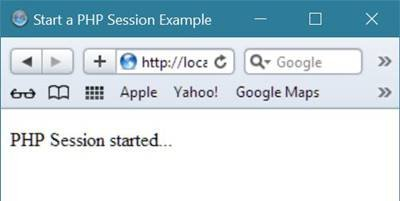As your PHP session is started after running the above PHP code. Now let's see how to set PHP session variables using the PHP global variable named \$_SESSION.

## PHP \$_SESSION Variable

The \$_SESSION is used to set session variable in PHP. Let's see how to set the value to a session variable using \$_SESSION in PHP.

```<?php
session_start();
?>
<!DOCTYPE html>
<html>
<title>PHP \$_SESSION Variable Example</title>
<body>
<?php
echo "<p>PHP Session started...</p>";
\$_SESSION['fname'] = 'Codes';
\$_SESSION['lname'] = 'Cracker';
// two php session variables are set
// with values, Codes and Cracker
echo "PHP Session Variables are Set";
?>
</body>
</html>```

If you run the above PHP \$_SESSION variable example code in your browser, then you will see the following output in your browser: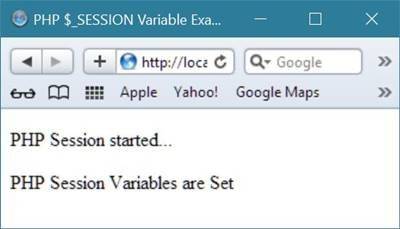Now let's see how to get the value of PHP session variable.

## Get Value of PHP Session Variables

Here is an example shows how to get the value of PHP session variables.

```<?php
session_start();
?>
<!DOCTYPE html>
<html>
<title>PHP \$_SESSION Variable Example</title>
<body>
<?php
echo "<p>PHP Session started...</p>";
echo "<p>My first name is: ".\$_SESSION['fname']."</p>";
echo "<p>My last name is: ".\$_SESSION['lname']."</p>";
echo "<p>My full name is: ".\$_SESSION['fname'].\$_SESSION['lname']."</p>";
?>
</body>
</html>```

Here is the sample output produced by the above PHP getting session variable's value example code: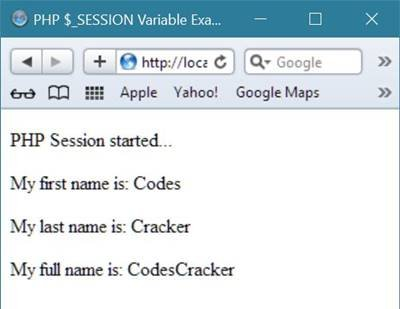Here is the modified version of the above example code illustrates how to get PHP session variable's value.

```<?php
session_start();
// isset is used to check whether
// the session variable named fname
// is set or not
if(isset(\$_SESSION['fname']))
{
// get and initialized the session variable to
// PHP variable named \$firstName
\$firstName = \$_SESSION['fname'];
// you can also type cast the variable's value
// as shown below, just for demo purpose
\$firstName = (string)\$firstName;
}
if(isset(\$_SESSION['lname']))
{
\$lastName = \$_SESSION['lname'];
}
?>
<!DOCTYPE html>
<html>
<title>PHP \$_SESSION Variable Example</title>
<body>
<?php
echo "<p>PHP Session started...</p>";
echo "<p>My first name is: ".\$firstName."</p>";
echo "<p>My last name is: ".\$lastName."</p>";
echo "<p>My full name is: ".\$firstName.\$lastName."</p>";
?>
</body>
</html>```

The above example code will produce the same output as of previous one.

## PHP session_destroy() Function

The session_destroy() function in PHP, used to destroy PHP session. Let's see how to destroy a PHP session using the function session_destroy().

## Delete/Destroy a PHP Session

Here is an example illustrating how to delete or destroy session using the function named session_destroy().

```<?php
session_start();
?>
<!DOCTYPE html>
<html>
<title>PHP Session Destroy Example</title>
<body>
<?php
echo "<p>PHP Session Destroying...</p>";
session_destroy();
echo "<p>PHP Session Destroyed successfully.</p>";
echo "<p>All the session variable along with its value are lost.</p>";
?>
</body>
</html>```

Here is the sample output of the above destroying session example code in PHP.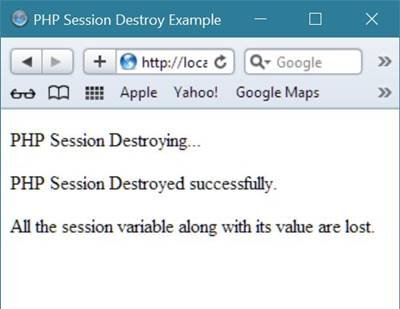Now, let's take some more examples on sessions in PHP.

## PHP Session Example

Here is an example of PHP session.

```<?php
session_start();
if(!isset(\$_SESSION['i']))
{
\$_SESSION['i'] = 1;
}
if(isset(\$_SESSION['i']))
{
\$i = \$_SESSION['i'];
}
?>
<!DOCTYPE html>
<html>
<title>PHP Session Example</title>
<body>
<?php
echo \$i;
\$i++;
\$_SESSION['i'] = \$i;
?>
</body>
</html>```

Here is the sample output of the above session example code in PHP.

After 1st run in your browser: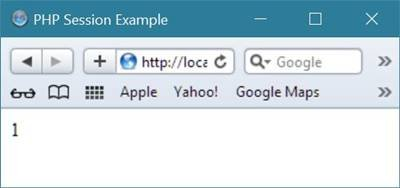After 2nd run in your browser, or after refreshing: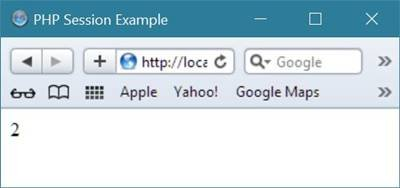After 3rd run in your browser, or again after refreshing: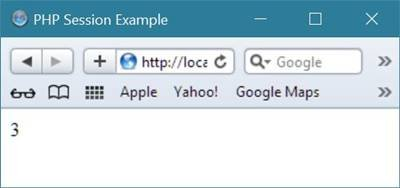The output will change, that is incremented by 1 when your refresh your browser.

Here is the modified version of the above PHP session example code:

```<?php
session_start();
if(!isset(\$_SESSION['i']))
{
\$_SESSION['i'] = 1;
}
if(isset(\$_SESSION['i']))
{
\$i = \$_SESSION['i'];
}
?>
<!DOCTYPE html>
<html>
<title>PHP Session Example</title>
<body>
<?php
echo \$i;
\$i++;
if(\$i==11)
{
session_destroy();
\$i = 1;
}
\$_SESSION['i'] = \$i;
?>
</body>
</html>```

Now when the value of the session variable named i becomes 11, then your session will be destroyed and the session variable is initialized with 1 as its value.

Here is the same PHP session example code which is more modified.

```<?php
session_start();
if(!isset(\$_SESSION['i']))
{
\$_SESSION['i'] = 1;
\$_SESSION['iOriginal'] = 1;
}
if(isset(\$_SESSION['i']))
{
\$i = \$_SESSION['i'];
\$iOriginal = \$_SESSION['iOriginal'];
}
?>
<!DOCTYPE html>
<html>
<title>PHP Session Example</title>
<body>
<?php
for(\$j=\$iOriginal; \$j<=\$i; \$j++)
{
if(\$j>1)
{
echo " - ";
}
echo \$j;
}
\$i++;
if(\$i==11)
{
session_destroy();
\$i = 1;
}
\$_SESSION['i'] = \$i;
?>
</body>
</html>```

Here is the initial output produced by the above session example code in PHP.

Initial output:Output after 1st refresh: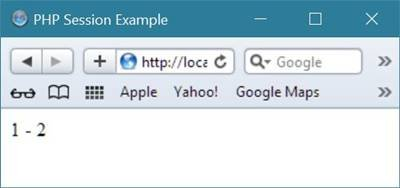Output after 3rd refresh: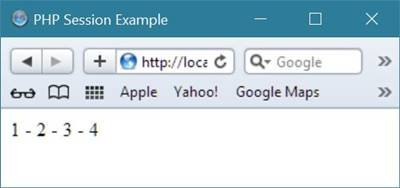Output after 9th refresh: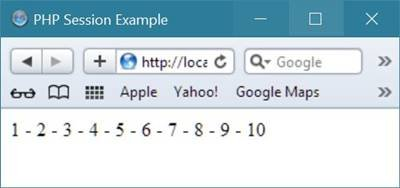Output after 10th refresh: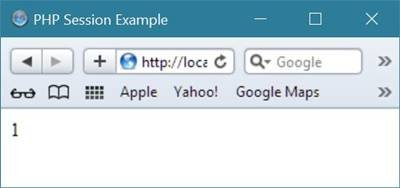Tools
Calculator